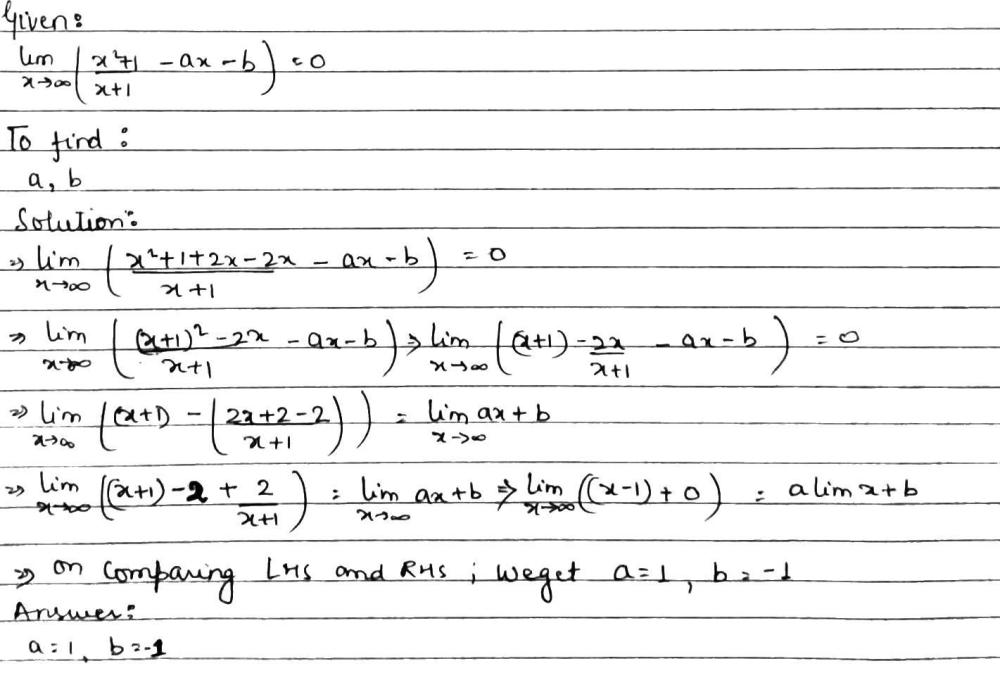Home/Class 11/Maths/

If $$\underset{x\xrightarrow{} \infty }{lim}\left(\frac{{x}^{2}+1}{x+1}-ax-b\right)=0$$then find values of a & bSpeed
00:00
05:57## QuestionMathsClass 11

If $$\underset{x\xrightarrow{} \infty }{lim}\left(\frac{{x}^{2}+1}{x+1}-ax-b\right)=0$$then find values of a & b

A=1,b=-1
4.64.6## Solution